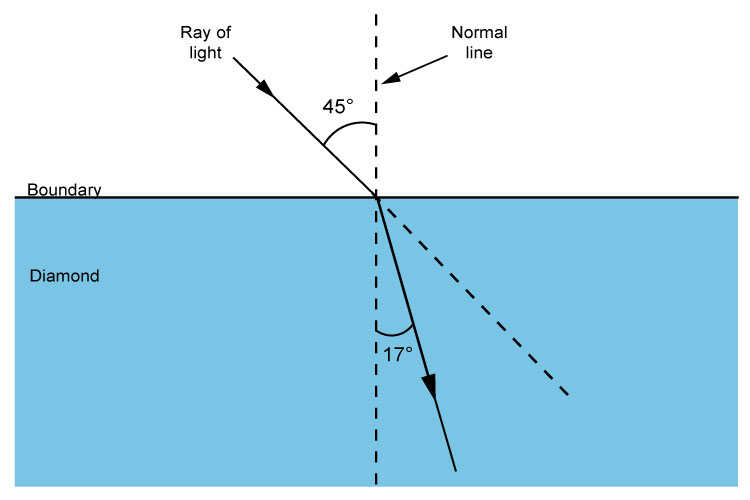# Different refractive indices

The refractive index is a ratio but it actually means that:

Refractive index (n) is the extent to which light is refracted (changes direction) when it enters a medium.

Also note that n is a constant for a particular medium.

Some examples are: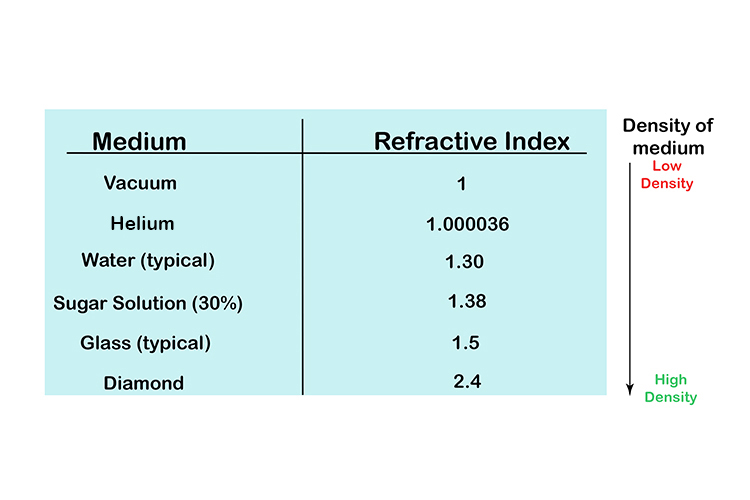NOTE: Low refractive index is low-density medium High refractive index is a high-density medium

So a high-refractive index (n) bends a lot more towards the normal line and a low refractive index (n) bends very little towards the normal line.

HIGH N° n = Much bend (HIGH bend) towards normal line
LOW N° n = Little bend (LOW bend) towards the normal line

Refraction of air to water n=1.3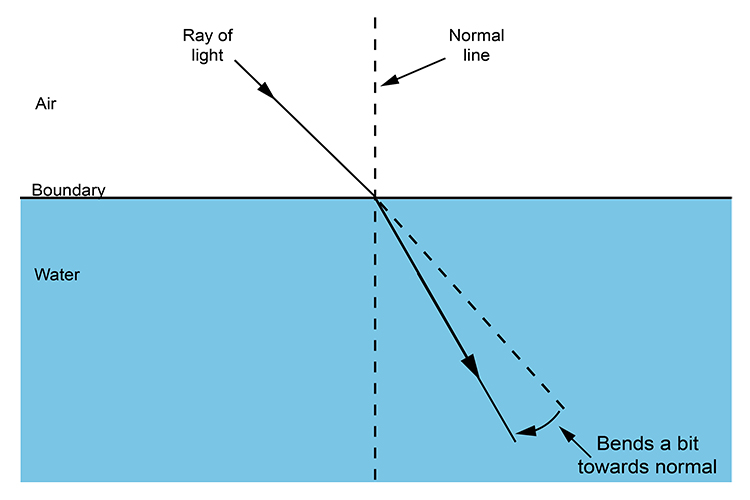Refraction of air to glass n=1.5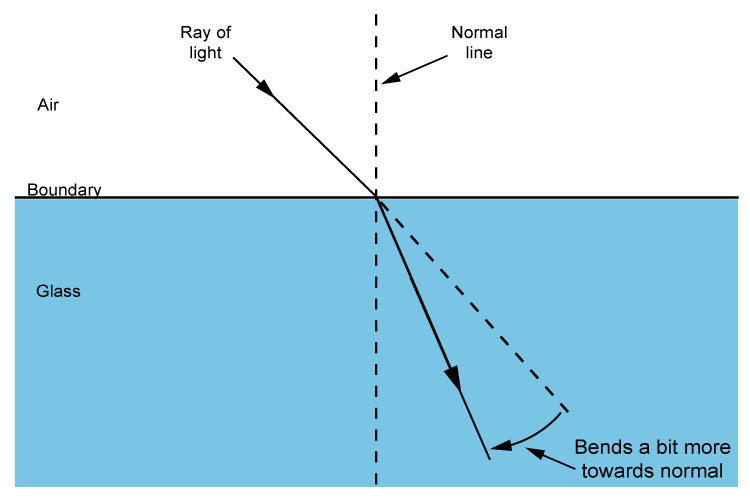Refraction of air to diamond n=2.4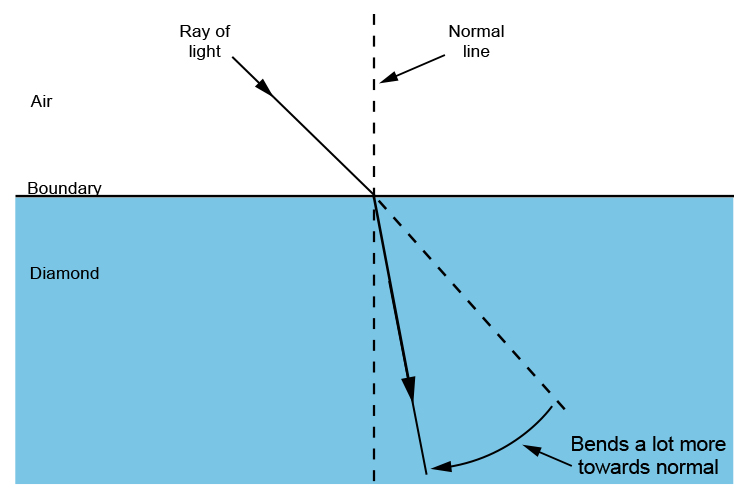We can then use this information to find any angle of refraction when we know the value n (refractive index) and angle of incidence (theta\i) for any light ray travelling from air to a thicker medium.

n=(sin\theta\i)/(sin\theta\r)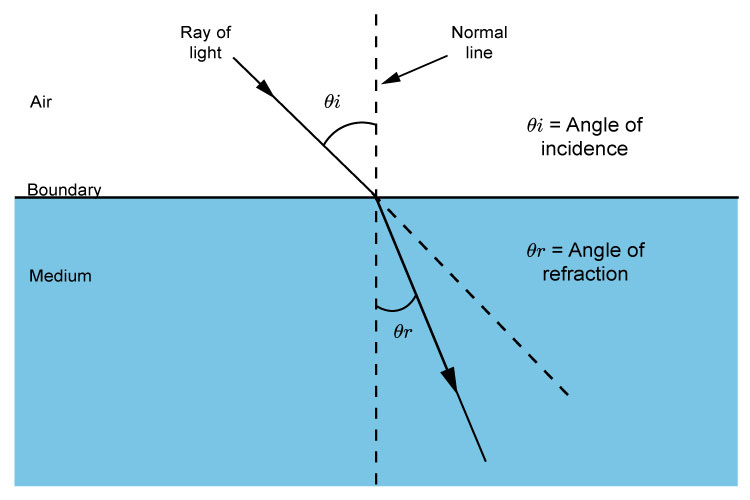## Refraction of air to water

n=1.3 and theta\i=45^circ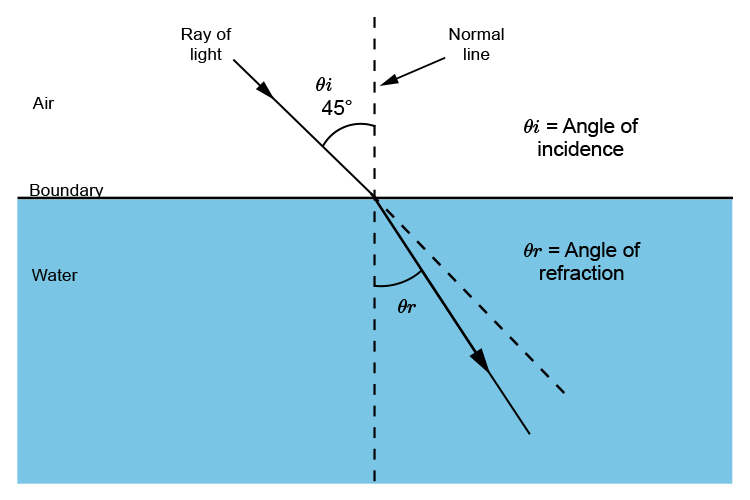n=(sin\theta\i)/(sin\theta\r)

1.3=(sin45)/(sin\theta\r)

Divide both sides by 1.3 and multiply both sides by sin\theta\r to get sin\theta\r on its own.

sin\theta\r=(sin45)/1.3

sin\theta\r=0.7071/1.3=0.5439

\theta\r=sin^(-1)\0.5439

\theta\r=32.95^circ~~33^circ

## Refraction of air to glass

n=1.5 and theta\i=45^circ

n=(sin\theta\i)/(sin\theta\r)

1.5=(sin45)/(sin\theta\r)

Divide both sides by 1.5 and multiply both sides by sin\theta\r to get:

sin\theta\r=(sin45)/1.5

sin\theta\r=0.7071/1.5=0.4714

\theta\r=sin^(-1)\0.4714

\theta\r=28.12^circ~~28^circ

## Refraction of air to diamond

n=2.4 and theta\i=45^circ

n=(sin\theta\i)/(sin\theta\r)

2.4=(sin45)/(sin\theta\r)

Divide both sides by 2.4 and multiply both sides by sin\theta\r to get:

sin\theta\r=(sin45)/2.4

sin\theta\r=0.7071/2.4=0.295

\theta\r=sin^(-1)\0.295

\theta\r=17.16^circ~~17^circ

## Summary

When light shines at 45° towards a surface the angle from the normal line to the refracted ray is:

 Water = 33° Glass = 28° Diamond = 17°

## Water 33°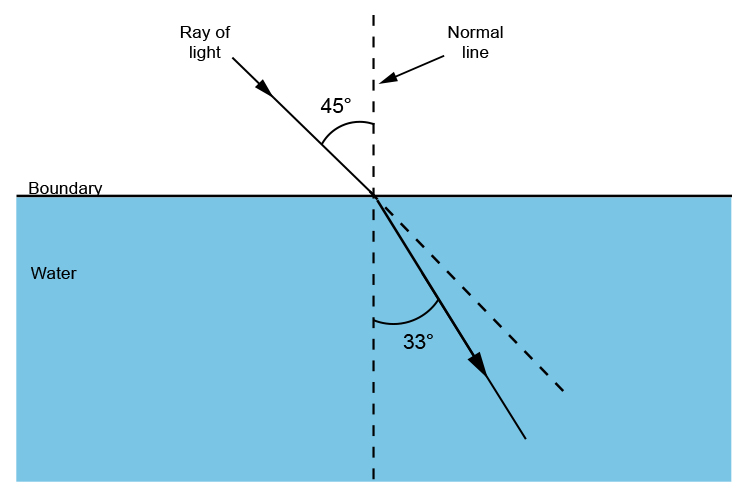## Glass 28°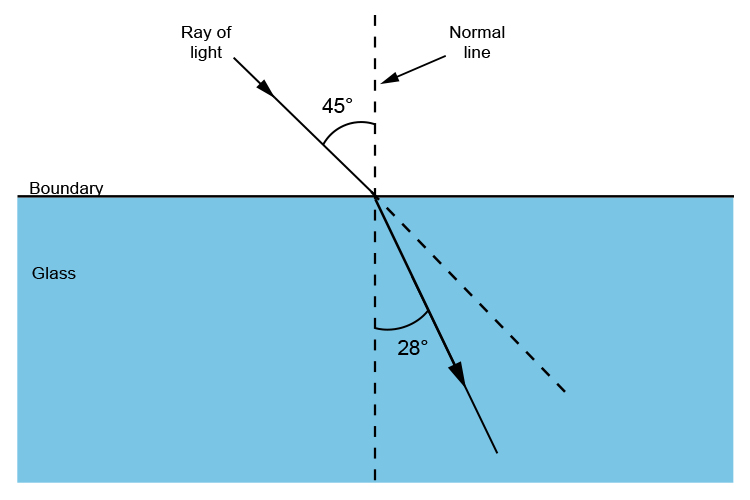## Diamond 17°